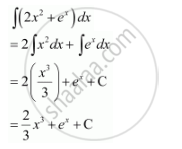Share

# Find the Following Integrals  Int(21x^2 + E^X)Dx - CBSE (Science) Class 12 - Mathematics

ConceptIntegration as an Inverse Process of Differentiation

#### Question

Find the following integrals  int(2x^2 + e^x)dx

#### SolutionIs there an error in this question or solution?

#### Video TutorialsVIEW ALL 

Solution Find the Following Integrals  Int(21x^2 + E^X)Dx Concept: Integration as an Inverse Process of Differentiation.
S